# 算法笔记-减治法

Posted by MaggicQ on March 27, 2017

# 减治法

• 减去一个常量：计算$a^n$的值，$a^n = a^{n-1} \times a$
• 减去一个常数因子: $a^n = a^{n/2} \times a^{n/2}$
• 减去的规模是可变的: 计算最大公约数的欧几里得算法，$gcd(m, n) = gcd(n, m mod n)$

## 插入排序## 拓扑排序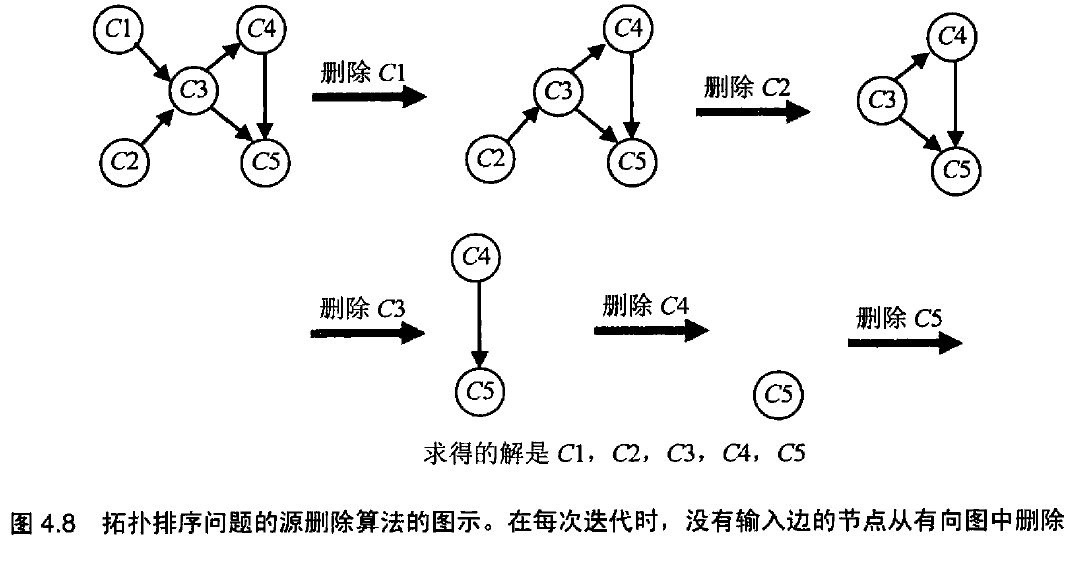## 生成组合对象的算法

### 生成排列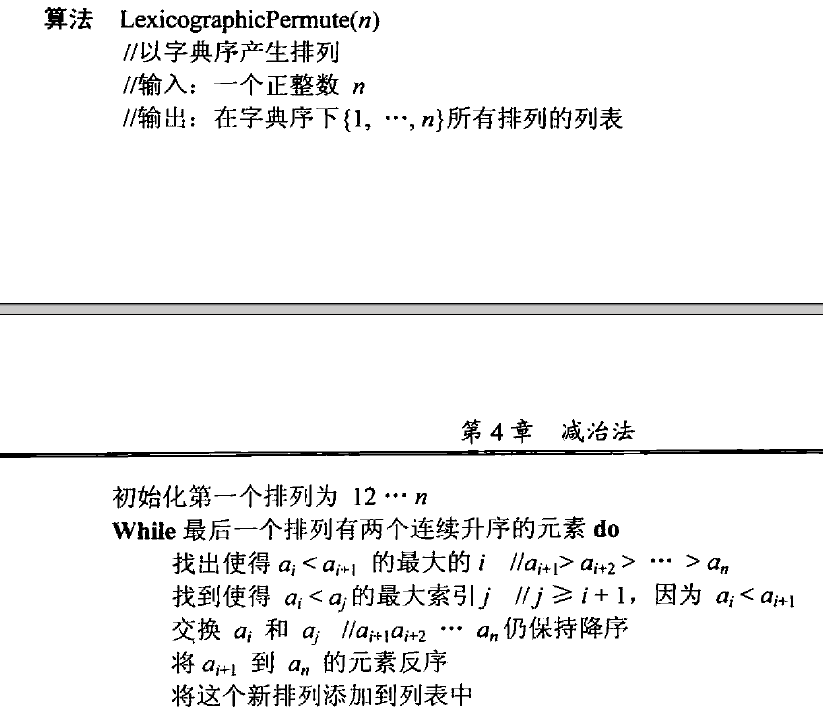## 减常因子算法

### 折半查找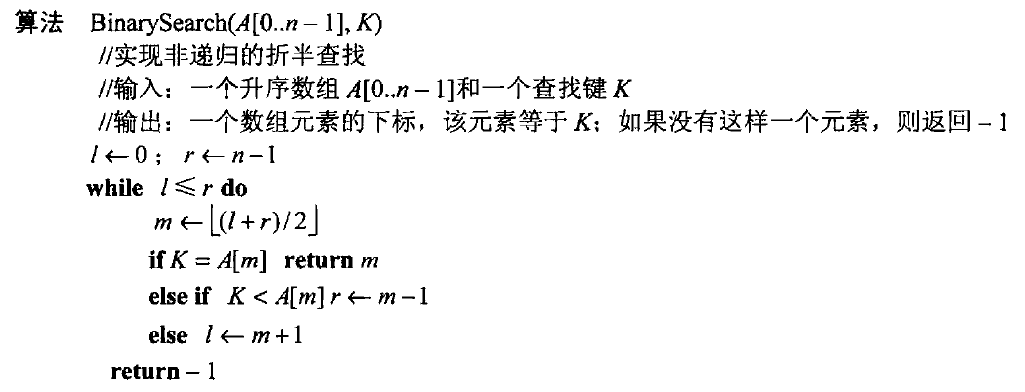### 假币问题

1.把硬币分为两堆

2.把硬币分为三堆，那么期望称重的次数大约会是 $log_3n$

### 俄式乘法

$n \times m = (n/2) \times (2m)$

$n \times m = (n-1)/2 \times (2m) + m$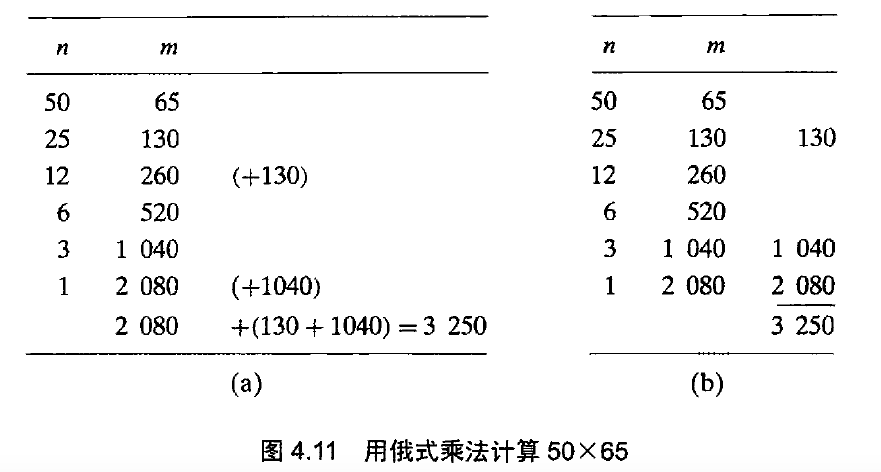### 约瑟夫斯问题

n为偶数的时候，$J(2k) = 2J(k) -1$

n为奇数的时候，$J(2k + 1) = 2J(k) + 1$

## 减可变规模算法

### 计算中值和选择问题

$k$个顺序统计量，当$k = \lceil n/2 \rceil$ 时，就是找中值的问题了。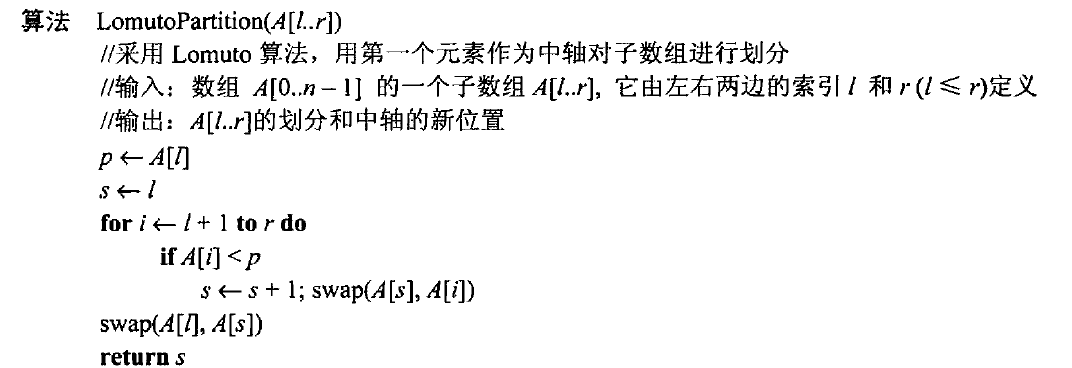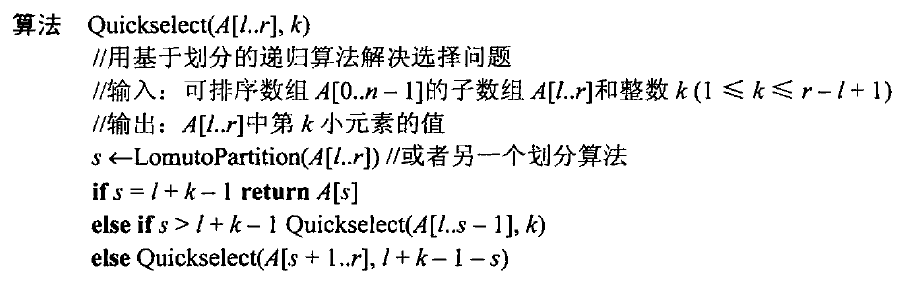### Nim游戏

$n = 0$ :败局

$1 \leq n \leq m$ : 胜局

$n = m+1$ ：败局，合法拿走任意数量的棋子都会把对方推入胜局

$m+2 \leq n \leq 2m+2$ : 胜局，走一步之后就可以留给对方 $m + 1$ 个棋子